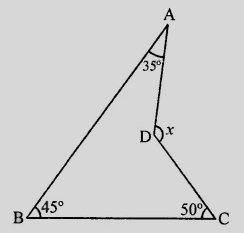"
">

# In the figure, compute the value of $x$."

To do:

We have to compute the value of $x$.

Solution:

In the given figure, $\angle ABC = 45^o, \angle BAD = 35^o$ and $\angle BCD = 50^o$.

Join $BD$ and produce it to $E$.In $\triangle \mathrm{ABD}$,

$\angle 1=\angle \mathrm{A}+\angle 3$..........(i)

In $\triangle \mathrm{CBD}$,

$\angle 2=\angle \mathrm{C}+\angle 4$......…(ii)

Adding equations (i) and (ii), we get,

$\angle 1+\angle 2=\angle \mathrm{A}+\angle 3+\angle \mathrm{C}+\angle 4$

$x=\angle \mathrm{A}+\angle 3+\angle 4+\angle \mathrm{C}$

$x=\angle \mathrm{A}+\angle \mathrm{B}+\angle \mathrm{C}$

$x=35^{\circ}+45^{\circ}+50^{\circ}$

$x=130^{\circ}$

The value of $x$ is $135^o$.

Updated on: 10-Oct-2022

29 Views